# 2.1 MAXWELL's Equations

MAXWELL's equations determine a relation among the electric and magnetic field, the space charge density and the current density. They read [4, p.31]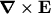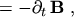(2.1)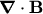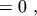(2.2)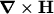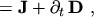(2.3)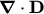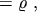(2.4)

where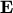and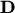are the electric field and displacement vectors, respectively,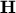and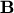are the magnetic field and induction vectors, respectively,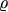is the total electric charge density, and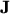the conduction current density.

Eqns. (2.1) and (2.2) and eqns. (2.3) and (2.4) each build a subsystem of their own. They are linked together by the relations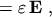(2.5)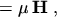(2.6)

where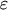and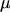are the permittivity and permeability, respectively. Eqn. (2.5) and eqn. (2.6) are valid only in materials where no piezoelectric or ferroelectric phenomena occur. In semiconductors the frequency dependence ofandcan be neglected.

Subsections

M. Gritsch: Numerical Modeling of Silicon-on-Insulator MOSFETs PDF